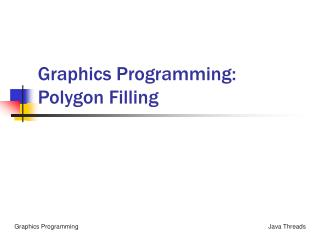DownloadDownload PresentationGraphics Programming: Polygon Filling

# Graphics Programming: Polygon Filling

Download Presentation## Graphics Programming: Polygon Filling

- - - - - - - - - - - - - - - - - - - - - - - - - - - E N D - - - - - - - - - - - - - - - - - - - - - - - - - - -
##### Presentation Transcript

1. Graphics Programming:Polygon Filling

2. Agenda • Terminology • Generalities • Scan-Line Polygon Fill Algorithm • Boundary-Fill Algorithm • Flood-Fill Algorithm

3. A Polygon • Vertex = point in space (2D or 3D) • + extra information in most cases • Polygon = ordered list of vertices • Each vertex connected with the next in the list • Last is connected with the first • Maybe more than one – polygons with holes • May contain self-intersections • Simple polygon – no holes or self-intersections • These are of most interest in CG

4. Polygons in graphics • The main geometric object used for interactive graphics • Efficient rendering algorithms exist • Polygon scanline rendering • Algorithms for making them look good exist • Lighting, shading, texture mapping • Lowest common denominator for all geometry • Can get close to any reasonable shape if enough of them

5. Drawing modes for polygons • Draw lines along polygon edges • Use algorithms e.g Bresenham • This is called wireframe mode • Draw filled polygons – new algorithms • Shaded polygons (shading modes) • Flat shaded – constant color for whole polygon • Gouraud shaded – interpolate vertex colors across the polygon

6. Polygon interior • Want to fill in (color) only pixels inside a polygon • What is “inside” of a polygon ? • Imagine a ray from the point to infinity • Count number of intersections with polygon edges • If N is odd, point is inside • If N is even, point is outside

7. Filling Polygons: Terminology Red arrow denotes a particular scan line. Blue disks denote span perimeter or ‘extrema’. Green disks denote other interior pixels in the span.

8. Filling Polygons: Generalities • Avoid drawing pixels more than once. 1. wastes computation 2. may cause unwanted color change if xor graphics context is used. 3. ugly artifacts can occur if two polygons share the same edge (e.g., dependence on drawing order). • Exploit coherence: pixels that are nearby each other tend to share common attributes (color, illumination, normal vectors, texture, etc.). • Span coherence: Pixels in the same scan line tend to be similar. • Scan-line coherence: Pixels in adjacent scan line tend to be similar. • Edge coherence: The corresponding span extrema in adjacent span lines tend to be near one another.

9. Interior Pixel Convention • Pixels that lie in the interior of a polygon belong to that polygon, and can be filled. • Pixels that lie on a left boundary or a lower boundary of a polygon belong to that polygon, and can be filled. • Pixels that have centers that fall outside the polygon, are said to be exterior and should not be drawn. • Pixels that lie on a right or an upper boundary do not belong to that polygon, and should not drawn.

10. Example 1 The boundary of a polygon: (In practice, a polygon is defined as a list of vertices.)

11. Example 2 Pixels closest to the polygon boundary – derived from Bresenham’s

12. Example 3 The scan extrema (blue) and interior (green) pixels that would be obtained if the span extrema were defined by the pixels closest to the boundary. Our convention excludes some of these.

13. Example 4 The scan extrema (blue) and interior (green) pixels that are obtained using our interior pixel convention for the given polygon (purple).

14. Basic Scan-Fill Algorithm Do the following for every scan line: 1. Compute the intersection of the current scan line with every polygon edge. 2. Sort the intersections in increasing order of the x coordinate. 3. Draw every pixel that lies between each pair of intersections, using a parity bit to designate the interior from the exterior. (Toggle the bit at each intersection.)

15. Polygon Fill B C Parity 0 = even 1 = odd Parity 0 1 0 D A E F

16. Polygon Fill 2 B C Parity 0 = even 1 = odd F D Parity 0 1 0 1 0 A E

17. Subtleties 1. The x-coordinates of the intersections are rounded towards the interior of the polygon. 2. If an intersection occurs precisely at an integer, following our convention, it is drawn if a piece of the polygon lies immediately above or to the right. 3. If a scanline intersects the polygon at a vertex, then only one of the intersections is counted in the parity test. To conform with our convention, the vertex is assumed to belong only to the edge that lies above it. (Edges, like spans, are regarded as intervals that are open at their maximum values and closed at their minimum values.) N.B., horizontal edges, and their vertices, are ignored completely. See: Rowe G. Computer Graphics with Java Chapter 1 pages 24 -34